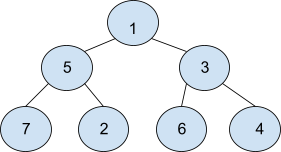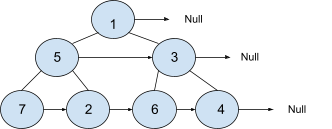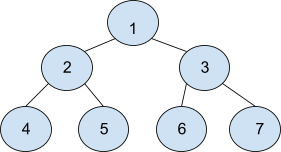# Populating Next Right Pointers In Each Node

Posted: 17 Mar, 2021
Difficulty: Moderate

## PROBLEM STATEMENT

#### Note :

``````1. A complete binary tree is a binary tree in which nodes at all levels except the last level have two children and nodes at the last level have 0 children.
2. Node ‘U’ is said to be the next node of ‘V’ if and only if  ‘U’ is just next to ‘V’ in tree representation.
3. Particularly root node and rightmost nodes have ‘next’ node equal to ‘Null’
4. Each node of the binary tree has three-pointers, ‘left’, ‘right’, and ‘next’. Here ‘left’ and ‘right’ are the children of node and ‘next’ is one extra pointer that we need to update.
``````

#### For Example :#### The‘next’ node for ‘1’ is ‘Null’, ‘2’ has ‘next’ node ‘6’, ‘5’ has ‘next’ node ‘3’, For the rest of the nodes check below.#### Input Format :

``````The first line contains an integer 'T' which denotes the number of test cases or queries to be run.

The first line of each test case contains elements of the tree in the level order form. The line consists of values of nodes separated by a single space. In case a node is null, we take -1 in its place.

For example, the input for the tree is depicted in the below image.
````````````1
2 3
4 5 6 7
-1 -1 -1 -1 -1 -1 -1 -1
``````

#### Explanation :

``````Level 1 :
The root node of the tree is 1

Level 2 :
Left child of 1 = 2
Right child of 1 = 3

Level 3 :
Left child of 2 = 4
Right child of 2 = 5
Left child of 3 =6
Right child of 3 =  7

Level 4 :
All children are ‘Null’

The first not-null node(of the previous level) is treated as the parent of the first two nodes of the current level. The second not-null node (of the previous level) is treated as the parent node for the next two nodes of the current level and so on.
The input ends when all nodes at the last level are null(-1).
``````

#### Note :

``````The above format was just to provide clarity on how the input is formed for a given tree.
The sequence will be put together in a single line separated by a single space. Hence, for the above-depicted tree, the input will be given as:
1 2 3 4 5 6 7 -1 -1 -1 -1 -1 -1 -1 -1
``````

#### Output Format :

``````For each test case, print the tree’s level order traversal after updating ‘next’ node for each node where  ‘-1’   denoting the null node.
The output of each test case will be printed in a separate line.
``````

#### Note :

``````You do not need to input or print anything, and it has already been taken care of. Just implement the given function.
``````

#### Constraints :

``````1 <= T <= 5
0 <= N <= 3000
1 <= data <= N
Where ‘data’ is the value of the node of the binary tree.
The given tree is always a Complete binary tree.

Time Limit: 1sec
``````Approach 1

The idea here is to Perform BFS while maintaining all nodes of the same level together, which can be done by storing nodes of the same level in a queue data structure, then for a particular level, we start popping nodes one by one and set the ‘next’ pointer of the previously popped node to the current node.

#### Example :

• Perform BFS  and maintain a queue for all nodes at the current level.
• Now, move to the next level and insert all nodes in the level to queue.
• Similarly, for the next level.

#### Algorithm:

• Declare a queue to store the nodes that are visited but not explored.
• Push root node in the queue.
• Explore Nodes until the queue is not empty.
• The queue contains all nodes in the same level.
• Declare a variable say ‘count’ equal to the current size of the queue.
• Declare a Variable ‘prev’ to store the previously popped node.
• Run a loop up to ‘count’ i.e. for all nodes from the current level.
• Pop the node from the queue and store it in a variable say ‘currNode’.
• If ‘currNode’ is not a left-most node. Set ‘prev->next’ = current popped node.
• ‘prev’ = ‘currNode’
• Push the child of ‘currNode’ in the queue.
• Finally, return the tree.